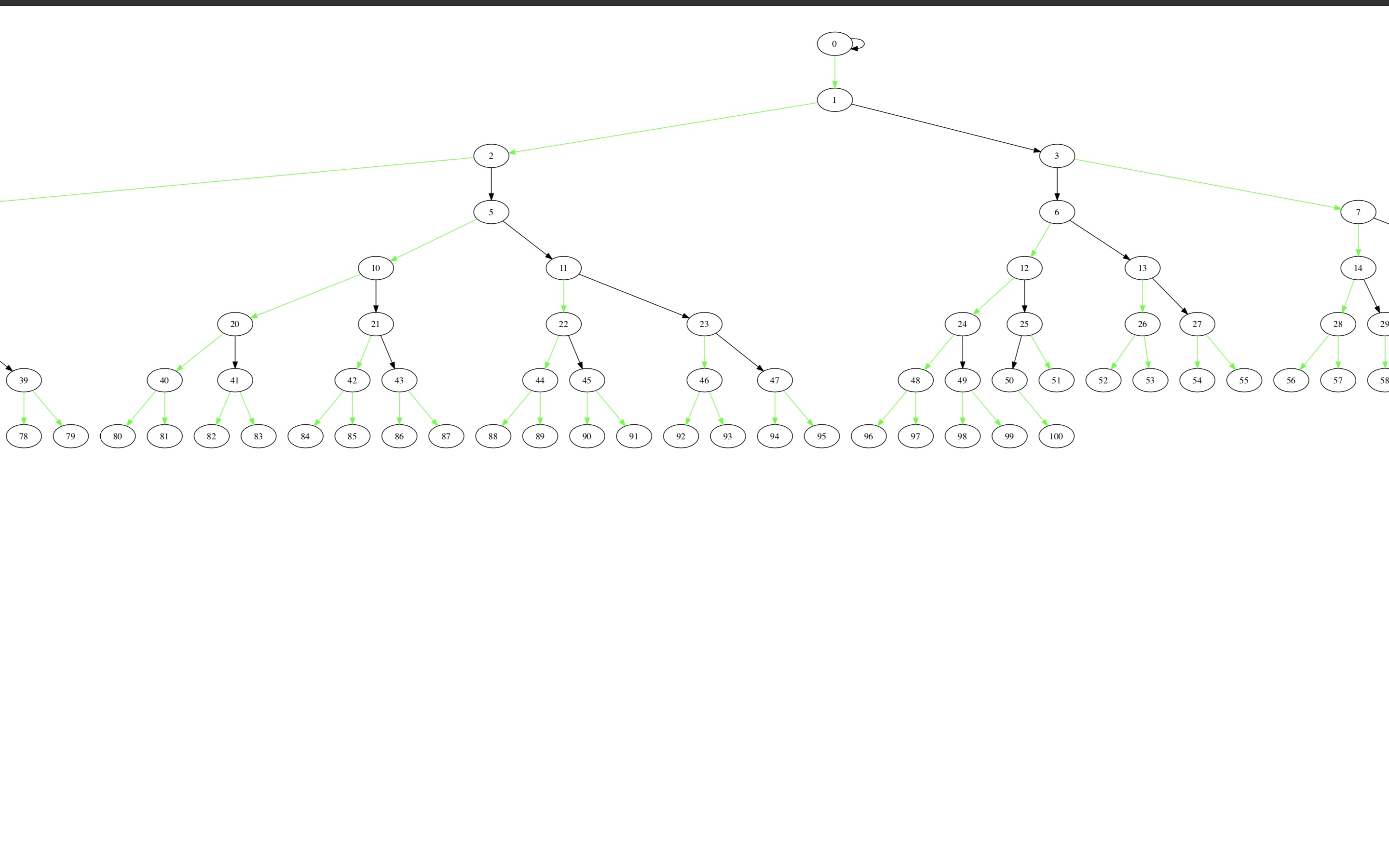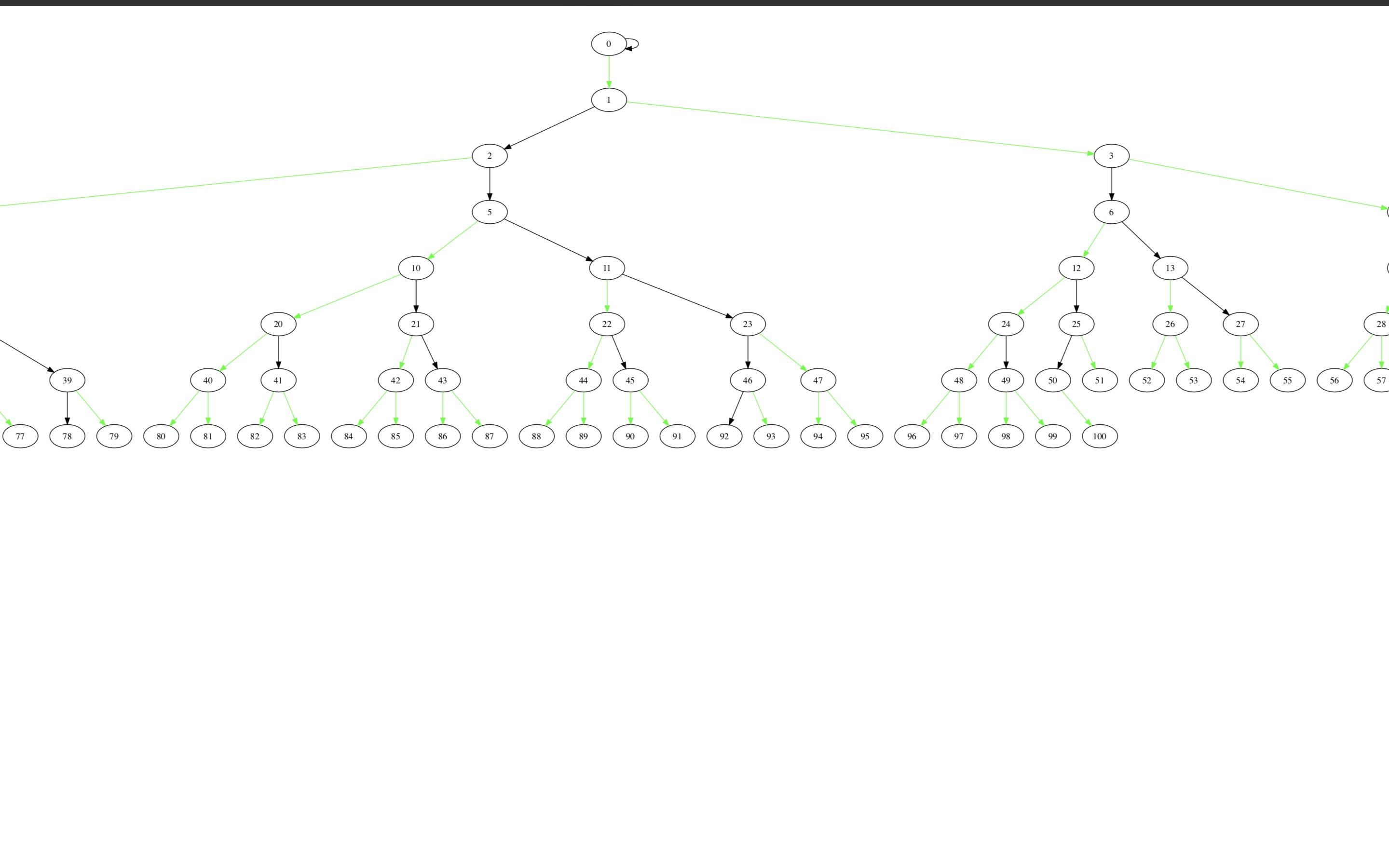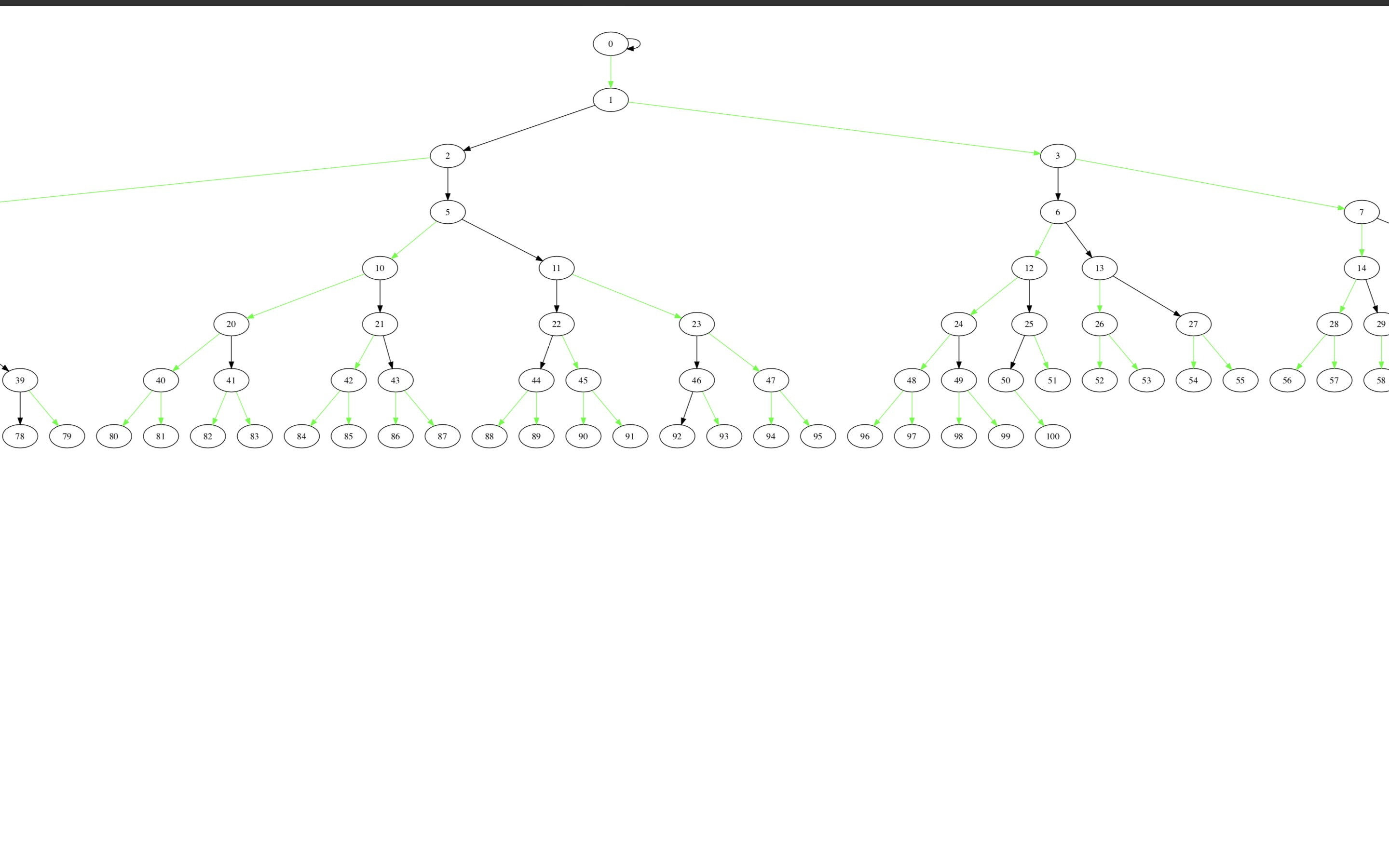# lct

在本质上他属于一种树剖分，将树剖成链，但是由于树是动态的，所以无法使用轻重剖分 ，因为重儿子可能会变化为轻儿子，轻儿子也可能变成重儿子，这里采取虚实剖分，按照虚链实链剖成链。

## 树的虚实剖分

一个节点最多有一个实儿子，当然他也可以不拥有实儿子，除实儿子以外，其他儿子 被称作轻儿子。于是我们就像轻重剖分一样，把这棵树给剖掉了。这样剖，特别武断，看似无法保证时间复杂度，然而并非如此。证明过程较难，此处不做 描述。下面三张图都是二叉堆的虚实剖分，黑色边为实链，绿色为虚链## 如何让被虚实剖分树具有动态

因为实儿子的选取限 制少，所以任何节点都有可能成为实儿子，当一棵树的形态发生了变化， 这并不影响我们的剖分，显然断边不会让我们的剖分出现任何问题，故而我们什么都不需要做，连边的话，无脑另此边为虚边即可。于是树的动态性不会影响 剖分

## 如何维护路径信息

此问题较复杂，我们先考虑根到某个节点的路径，我们是这样做的，变换虚实边，使得 经过根的实链的两个端点分别是根和那个节点。可以证明，这样的剖分存在。变换虚实边之后，那条路径就被我们剖了出来，链是由数据结构来维护的， 于是我们就可以解决该路径问题了。
对于一般路径而言,我们直接强制换根来解决

## 我们该选取何种数据结构

先来总结一下操作
操作1:把实边变为虚边,对应到链上 就是把一条链切断，在数据结构中体现为分裂
操作2:把实边变为虚边,对应到链上 就是把两条链合并，在数据结构中体现为合并
操作3:换根？这个较复杂，暂时不考虑
操作4:路径询问，在数据结构中体现为 整个数据结构维护的序列的性质，也即全段区间询问。
操作5:路径修改，在数据结构中体现为 整个数据结构维护的序列的性质，也即全段区间修改。
splay可以胜任，它支持分裂合并以及区间询问、修改,如果我们把整条链放入splay,用其中序遍历结果来 维护链，每一个splay再记录一个父亲，也即是此splay维护的链的父亲是谁？定义:链的父亲为链上深度最小的节点的父亲。，可以 证明，这些信息合并起来，对应到树是唯一的，于是问题基本解决
现在我们来考虑换根，换根是怎么一回事?我们来看上文的最后一张图，根为1，如果我们想把根换为44，怎嘛办呢？ 如果你足够聪明，是可以想到做法的，实际上我们只需要将链1->2->5->11->22->44反转即可。证明过程很简单，此处不做证明。
至此，lct流程基本阐述完成，后面讲解细节操作。

## 核心操作access

函数access(x)，被调用后，我们会让起点在根，终点在x的链剖出，此链为过根节点的实链。操作很简单，我们 先将x伸展到它所在的那个splay的根，然后将它和他的右子树断开，为什么呢？因为他的右子树绝不在我们要的实链上，然后将它合并到此链的父亲上，但是此链的父亲可能已经有了 实儿子，那我们就将它断开，然后在把x子树合并上去，虽然是这么说的但是我们实际代码确实先合并子树，然后断链，其实是一样的。然后对其父亲作此操作，就结束了

## 后面的其他没啥技术含量，也特别简单，就不讲了，代码如下

```struct Link_Cut_Tree{
static const int N=1e5+555;
int top,c[N],fa[N],xr[N],sta[N],rev[N],val[N];
void init(int n){
memset(fa,0,n<<2);
memset(rev,0,n<<2);
memset(c,0,n<<3);
}
inline void pushup(int x){xr[x]=xr[c[x]]^xr[c[x]]^val[x];}
inline void pushdown(int x){
int l=c[x],r=c[x];
if(rev[x]){
rev[l]^=1;rev[r]^=1;rev[x]^=1;
swap(c[x],c[x]);
}
}
inline bool isroot(int x){return c[fa[x]]!=x&&c[fa[x]]!=x;}
void rotate(int x){
int y=fa[x],z=fa[y],xis=c[y]==x,yis=c[z]==y;//
if(!isroot(y))c[z][yis]=x;//son
fa[x]=z;fa[y]=x;fa[c[x][xis^1]]=y;//father
c[y][xis]=c[x][xis^1];c[x][xis^1]=y;//son
pushup(y);pushup(x);
}
void splay(int x){
top=1;sta[top]=x;//init stack
for(int i=x;!isroot(i);i=fa[i])sta[++top]=fa[i];//update stack
while(!isroot(x)){
int y=fa[x],z=fa[y];
if(!isroot(y)){
if((c[y]==x)^(c[z]==y))rotate(x);
else rotate(y);
}rotate(x);
}
}
void access(int x){for(int t=0;x;t=x,x=fa[x])splay(x),c[x]=t,pushup(x);}
int treeroot(int x){access(x);splay(x);while(c[x])x=c[x];return x;}
void makeroot(int x){access(x);splay(x);rev[x]^=1;}
void cut(int x){access(x);splay(x);fa[c[x]]=fa[x];c[x]=0;fa[x]=0;}//cut the subtree x and let x to be the root